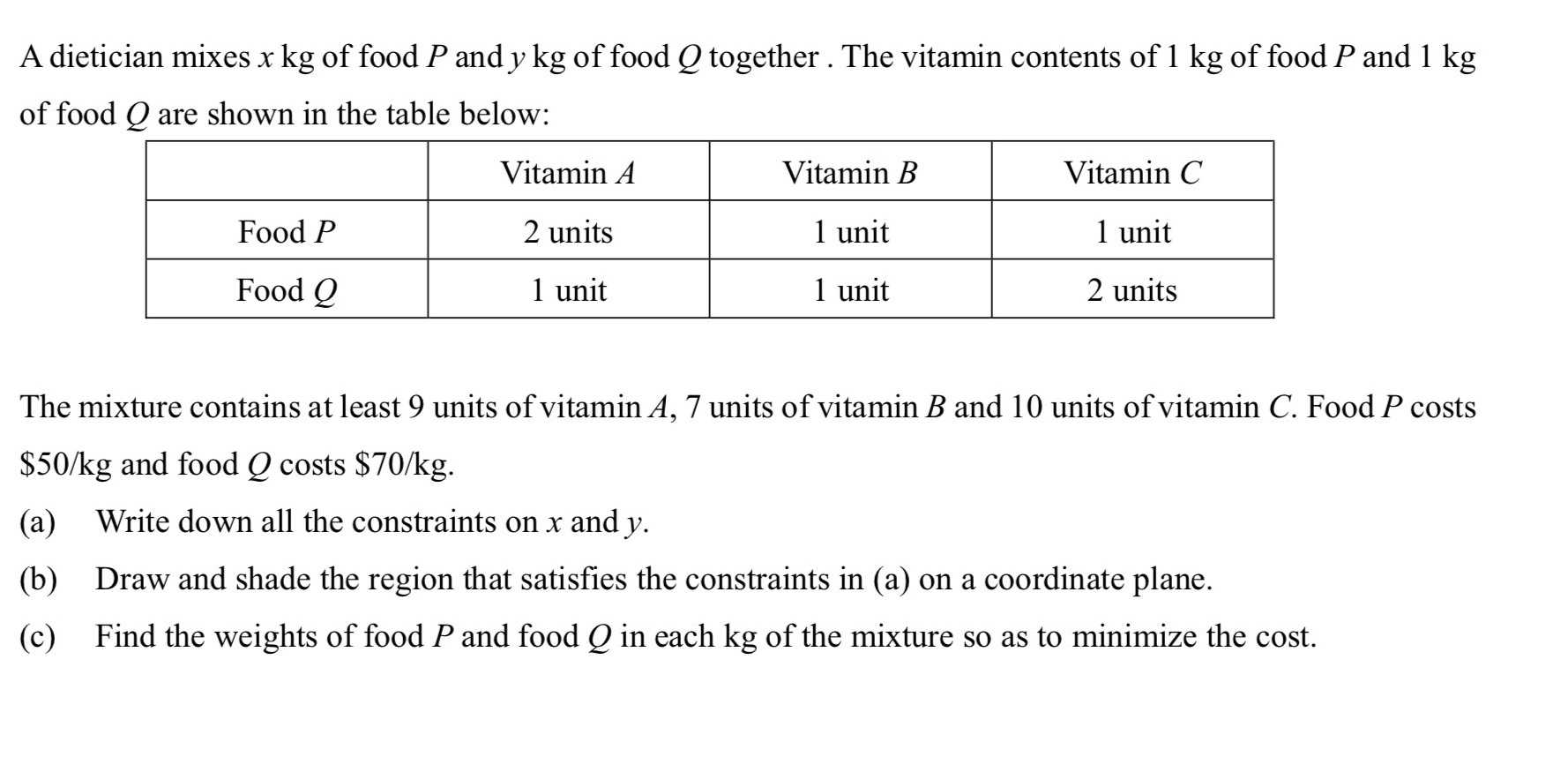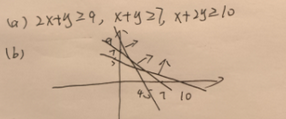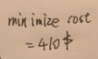### ¿Todavía tienes preguntas de matemáticas?

Pregunte a nuestros tutores expertos
Algebra
PreguntaA dietician mixes $$x kg$$ of food $$P$$ and $$y kg$$ of food $$Q$$ together. The vitamin contents of $$1 kg$$ of food $$P$$ and $$1 kg$$ of food $$Q$$ are shown in the table below:

The mixture contains at least $$9$$ units of vitamin $$A , 7$$ units of vitamin $$B$$ and $$10$$ units of vitamin $$C$$ . Food $$P$$ costs $$\ 50 / kg$$ and food $$Q$$ costs $$\ 70 / kg$$ .

(a) Write down all the constraints on $$x$$ and $$y$$ .

(b) Draw and shade the region that satisfies the constraints in (a) on a coordinate plane.

(c) Find the weights of food $$P$$ and food $$Q$$ in each kg of the mixture so as to minimize the cost.